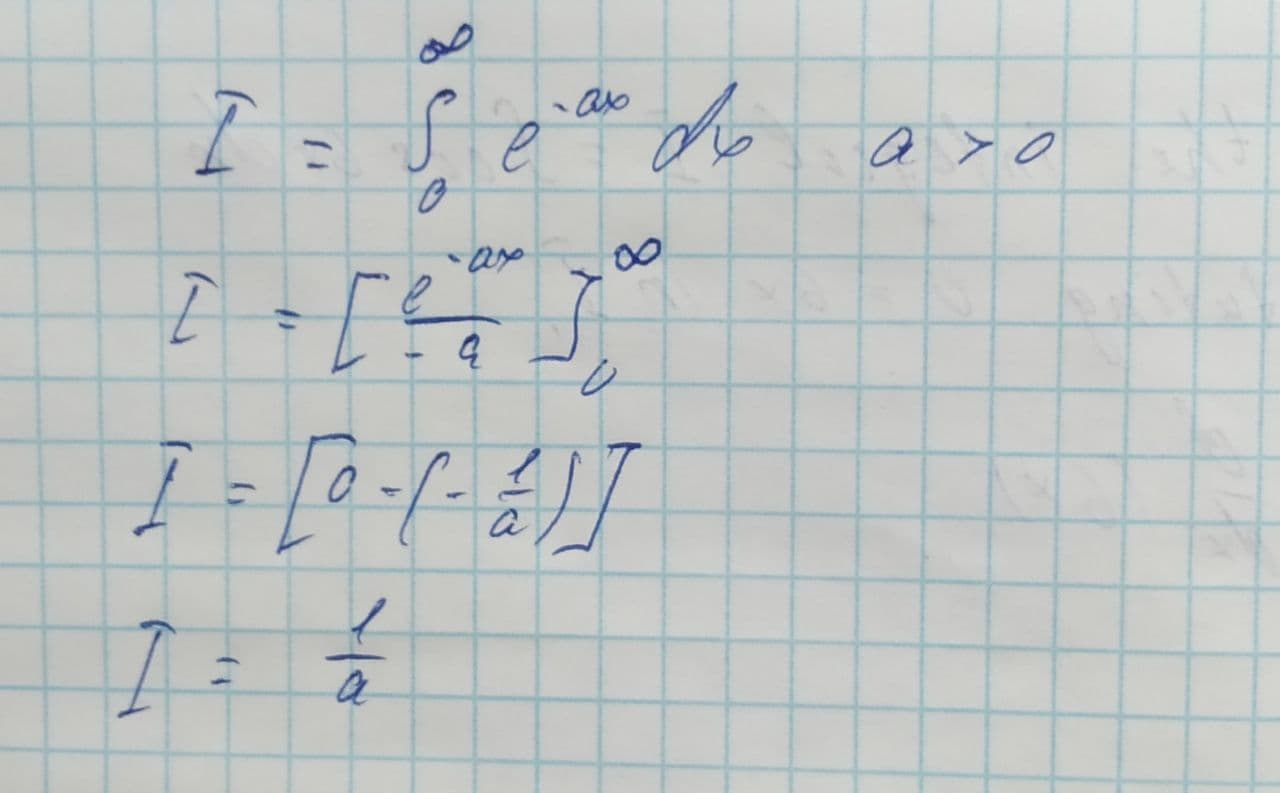# Evaluate the following integrals or stat that they diverge \int_{0}^{\infty}e^{-ax} dx, a>0tricotasu 2021-08-14 Answered
Evaluate the following integrals or stat that they diverge.
${\int }_{0}^{\mathrm{\infty }}{e}^{-ax}dx,a>0$
You can still ask an expert for help

• Questions are typically answered in as fast as 30 minutes

Solve your problem for the price of one coffee

• Math expert for every subject
• Pay only if we can solve ityunitsiL
Step 1
Any integral is an improper integral if it follows these two conditions:
1) One of the limit of the integral reaches to infinity.
2) The function whose integral has to be calculated has to be unbounded. This means that it should not be ending at a point. It has to be everlasting.
The integral of these types have no special rule to calculate. These are just like normal integral.
Integration is done below:
Step 2